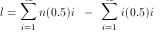Click to Get updated NTA UGC NET CS Test Series           Study Material for UGC NET Computer Science- 2019

# Computer Networks - Protocols

21:

Adaptive or dynamic directory used in packet routing changes

 A. within each user session B. with each user session C. at system generation time only D. both (a) and (b) Answer Report Discuss Option: A Explanation : Click on Discuss to view users comments. Write your comments here:
22:

A networking system has an n layers. Application generated message of length M bytes. At each of the layer, a h-byte header is added, what fraction of the network bandwidth is filled with headers ?

 A. M / (n x M) bytes B. h x n x M bytes C. (n x h) / M bytes D. M / (n x m) Answer Report Discuss Option: C Explanation : Click on Discuss to view users comments. Write your comments here:
23:

Let the clock-driven scheme for generating initial sequence numbers is used with a 15-bit wide clock counter. The clock ticks once every 100 msec, and the maximum poacket lifetime is 60 sec.
How often need resynchronization take place in the worst case ?

 A. 2216.8 sec B. 2816.8 sec C. 3216.8 sec D. 3416.8 sec Answer Report Discuss Option: C Explanation : The clock takes 32768 ticks, i.e., 32768 sec to cycle around. At zero generation rate, the sender would enter the forbidden zone at                                   = 3276.8 - 60                                   =  3216.8 sec.  Click on Discuss to view users comments. Write your comments here:
24:

For a 1-Gbps network operating over 4000 km, the delay is the limiting factor, not the bandwidth. Consider a MAN with the average source and destination 20 km apart. At what data rate does the round-trip delay due to the speed of light equal the transmision delay for a1-KB packet ?

 A. 24 Mbps B. 36 Mbps C. 40 Mbps D. 48 Mbps Answer Report Discuss Option: C Explanation :  Speed  ight in fiber and copper = 200 km/msec. For a 20-km line, the delay is 100 μsec one way and 200 μsec round trip. A 1 KB packet has 8192 bits. If the time to send 8192 bits and get the acknowledgement is 200 μsec, the transmission and propagation delays are equal.  If B is the bit time, then         8192 = 2 x 10-4 sec.         Data rate, 1 / B = 40 Mbps. (approx)  Click on Discuss to view users comments. Write your comments here:
25:

A group of 2n - 1 routers are interconnected in a centralised binary tree, with a router at each tree node. Router i communicates with router j by sending a message to the root of the tree. The root then sends the message back down to j. The mean number of hops per message for large n, assuming that all router pairs are equally likely will be

 A. n - 4 B. 2n - 1 C. n - 2 D. 2n - 4 Answer Report Discuss Option: D Explanation : Mean router-router path is twice the mean router-root path. Number levels of the tree with the root as 1 and the deepest level as n. Path from the root to level n requires n - 1 hops, and 0.50 of the routers are at this level. Path from root to level n - 1 has 0.25 of the routers, and a length of n – 2 hops. therefore,  Mean path length l = 0.5 x (n-1) + 0.25 x (n-2) + 0.125 x (n-3) +....    The expression reduces to l = n – 2 Mean router-router path = 2n – 4.Click on Discuss to view users comments. Write your comments here:X